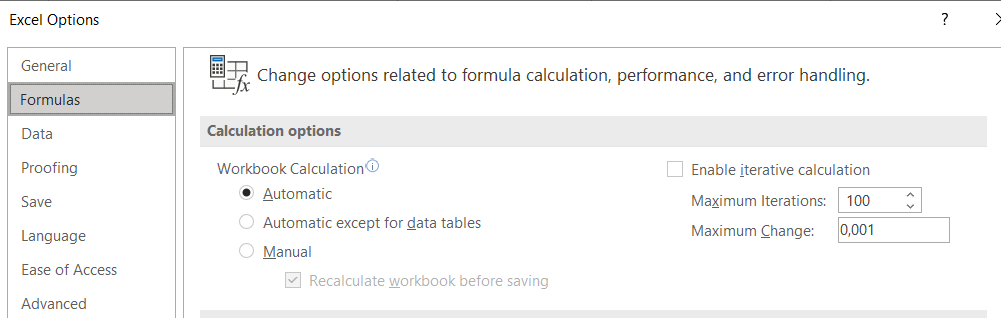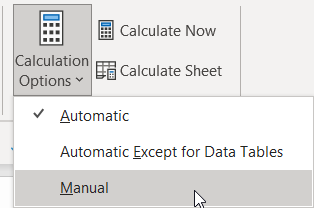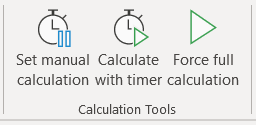# Excel calculation options and shortcuts

Calculation Options

If you have a large workbook with lots of lookup formulas or complex calculations the whole workbook can be slow to recalculate so you can turn on the manual calculation option.

There are two ways to do this:

1. File, Options, Formulas2. Formulas ribbon, Calculation, Calculation Options (click the down arrow to open the dropdown)In each case you will see three or four options:

• Automatic (standard setting) - generally recommended so that you can see the effects of spreadsheet changes immediately.

• Automatic except for data tables - recommended if you have data tables in your spreadsheet. Data tables are great for calculating the results of different scenarios to compare them but require multiple calculations of your model so this option is highly recommended in such cases to avoid slowing your model down unnecessarily.

• Manual - recommended for large and slow workbooks - this makes it much easier to work with your file but you can't see the effects of changes immediately, hence you need to manually initiate a recalculation (see below).

• Manual: Recalculate workbook before saving - this extra option is only available via File, Options. I recommend that you turn this off if you save your file often (e.g., so that in the case of a crash you have a recent copy of your file) but do not want Excel to recalculate your file each time as this can take a while with large, slow models.

Under File, Options you also get options for iterative calculations. This can be turned on if you have logical circular references on your model e.g., interest costs affect the closing bank balance which then affects the interest costs. The standard settings (100 iterations, maximum change 0.001) are generally good but, if necessary, you can increase the number of iterations and/or reduce the maximum change. Note that the accuracy here also appears to be used for Goal Seek calculations, so you can also make such changes to improve the accuracy of goal seek results.

Recalculation options and shortcuts

If you are using the manual calculation option, then you will have to manually start a recalculation at a suitable point in time (e.g. when you have completed a new model section). Here there are also a few options, some available with the ribbon/mouse, others with keyboard shortcuts as follows.

• Calculate now (shortcut F9) - recalculates all open workbooks. Close unnecessary files to speed this up.

• Calculate sheet (shortcut Shift F9) - recalculates only the currently active sheet. This is quicker than recalculating the whole workbook if you only need to update/check a single sheet.

• Calculate a single cell (shortcut only: F2 followed by the enter key) - recalculates only the selected cell. This is the quickest option, but only a single cell, useful on large sheets where even a calculate sheet takes a while. If you select a block of cells, you can press F2 and enter repeatedly to calculate each one in turn, Excel then jumps to the next cell.

• Force full recalculation (shortcut only: Ctrl Shift Alt F9) – this can be useful on the rare occasions when model results do not appear to reflect recent changes you have made. To reduce calculation time Excel normally only recalculates dependents of cells which have changed (and their dependents etc.) plus all volatile functions such as TODAY, INDIRECT and OFFSET. Normally this works very well. Very occasionally however, it can be useful to force a full recalculation. This can take a while, so close unnecessary files before you start.